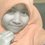# arithmetic

Which number should come next in this series?

25,24,22,19,15

A. 4 B. 5 C. 10 D. 14Note by Pryhant Kielh
6 years, 9 months ago

This discussion board is a place to discuss our Daily Challenges and the math and science related to those challenges. Explanations are more than just a solution — they should explain the steps and thinking strategies that you used to obtain the solution. Comments should further the discussion of math and science.

When posting on Brilliant:

• Use the emojis to react to an explanation, whether you're congratulating a job well done , or just really confused .
• Ask specific questions about the challenge or the steps in somebody's explanation. Well-posed questions can add a lot to the discussion, but posting "I don't understand!" doesn't help anyone.
• Try to contribute something new to the discussion, whether it is an extension, generalization or other idea related to the challenge.

MarkdownAppears as
*italics* or _italics_ italics
**bold** or __bold__ bold
- bulleted- list
• bulleted
• list
1. numbered2. list
1. numbered
2. list
Note: you must add a full line of space before and after lists for them to show up correctly
paragraph 1paragraph 2

paragraph 1

paragraph 2

[example link](https://brilliant.org)example link
> This is a quote
This is a quote
    # I indented these lines
# 4 spaces, and now they show
# up as a code block.

print "hello world"
# I indented these lines
# 4 spaces, and now they show
# up as a code block.

print "hello world"
MathAppears as
Remember to wrap math in $$ ... $$ or $ ... $ to ensure proper formatting.
2 \times 3 $2 \times 3$
2^{34} $2^{34}$
a_{i-1} $a_{i-1}$
\frac{2}{3} $\frac{2}{3}$
\sqrt{2} $\sqrt{2}$
\sum_{i=1}^3 $\sum_{i=1}^3$
\sin \theta $\sin \theta$
\boxed{123} $\boxed{123}$

Sort by:

Although the intended answer here is $C$, you should know that there is no correct answer to this. I could easily say the answer is $B$.

You can ask me why. Well, my explanation is: the $n ^{th}$ term of this sequence is $f(n)$ where $f(n)=-\frac{n^5-15n^4+85n^3-213n^2+262n-720}{24}.$Plug in $n=1, 2, 3, 4, 5$ and get $f(n)= 25, 24, 22, 19, 15$ [your sequence]. Plug in $n=6$ and you'll get $f(n)=5$. So, $B$ is a perfectly valid answer.

I know that this is not the answer you want and you're probably getting angry at me right now! What I'm trying to say is there's no correct answer.Alt text

- 6 years, 9 months ago

How did u get this f(n)

- 6 years, 9 months ago

why was that comment deleted i wrote in it that tushar is a cheater who cheats from many id's and has atleast 20 ids on brillaint

- 6 years, 9 months ago

Tushar's many accounts have been banned. Please in the future email me at discussions@brilliant.org to report cheating. I deleted the comment because, I thought it distracted from the point Mursalin was trying to make.

Staff - 6 years, 9 months ago

I used Lagrange interpolation. But this is not the only way.

For example: you could add $(n-1)(n-2)(n-3)(n-4)(n-5)$ to the polynomial to get a different value for the sixth term.

This way you can practically get anything (even complex numbers) for the sixth term!

- 6 years, 9 months ago

The $n^{th}$ term of this series is $A_{n}=A_{n-1}-n$ where $25$ is the $0th$ term; hence the $5^{th}$ term which is what your looking for is $A_{5}=A_{4}-5$. Therefore $A_{5}=15-5$ which is $10$ hence the answer is $C$.

- 6 years, 9 months ago

10, because 25-1=24, 24-2=22,22-3=19,19-4=15, so 15-5=10

- 5 years, 8 months ago

10

- 5 years, 5 months ago

10

- 4 years, 10 months ago

we can do this in this way to: here consecutive nos are being subtracted.i from 25 then 2 from 24 and then 3 from 22 and so on..........

so the ultimate answer is 15-5(as it is the 5th term in sequence)=10 (c)

- 6 years, 9 months ago

C,10

- 6 years, 9 months ago

Well, It is necessary to ask you what part of Mathematics is it after we will conclude an answer.

- 6 years, 9 months ago

10

- 6 years, 9 months ago

c

- 6 years, 9 months ago

It's C. 10

- 6 years, 9 months ago